GEATbx: Main page  Tutorial  Algorithms  M-functions  Parameter/Options  Example functions  www.geatbx.com

GEATbx: Example Functions (single and multi-objective functions) 1 Introduction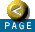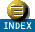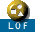1 Introduction

This document describes a number of test functions implemented for use with the Genetic and Evolutionary Algorithm Toolbox for Matlab (GEATbx). These functions are drawn from the literature on evolutionary algorithms and global optimization. The first Section describes a set of common parametric test problems implemented as Matlab m-files. The second Section presents a number of dynamic systems, implemented in Simulink, as s-files and m-files as appropriate.

1.1 Examples of Parametric Optimization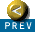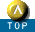Each of the functions in Chapter 2 is described by the function definition, one or more 3-D graphics to show the properties of the function and a description of the features of the function.

1.2 Examples of Multi-objective Optimization

The functions in Chapter 3 constitute multi-objective example functions. For each of them the definition and a description of the features of the function are given. Plots of the PARETO-front in search and solution space enhance the understanding of the functions. If useful, 3-D graphics showing the search space are provided.

All of the test function implementations are scaleable, i.e. the functions can be called with as many dimensions as necessary and the default dimension of the test functions is adjustable via a single parameter value inside the function.

For writing own objective functions see Writing objective functions.GEATbx: Main page  Tutorial  Algorithms  M-functions  Parameter/Options  Example functions  www.geatbx.com

This document is part of version 3.8 of the GEATbx: Genetic and Evolutionary Algorithm Toolbox for use with Matlab - www.geatbx.com.
The Genetic and Evolutionary Algorithm Toolbox is not public domain.